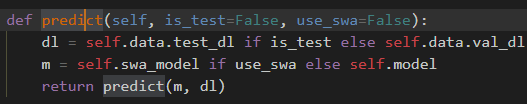# Recursion in Fastai library

I don’t understand how this Recursion workThat’s because it isn’t recursion. It’s calling the predict function from model.py

``````def predict(m, dl):
preda,_ = predict_with_targs_(m, dl)
return np.concatenate(preda)``````
1 Like

How can I find where this function defined?

Line 248 of model.py